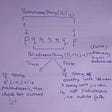# String Compression

`const stringCompression = (string) => {let str = string.split("");let count = 0;let hash = {};let result = "";}`
`for (let i = 0; i < str.length; i++) {}`
• If the current iteration does NOT exist in the hash, store it in the hash = to itself.
• Then, if the current iteration and the next are not the same, increment the count variable, add the current letter to the result variable + the count, and set the count = 0.
• Else, increment the count
`for (let i = 0; i < str.length; i++) {if (!hash[str[i]]) {hash[str[i]] = str[i];//first bullet point ^if (str[i] !== str[i + 1]) {count++;result += str[i] + count;count = 0;//second bullet point ^} else {count++;//third bullet point ^}`
• If the current iteration DOES exist in the hash and…
• If the current iteration and the next are not the same, increment the count variable, add the current letter to the result variable + the count, and set the count = 0.
• Else, increment the count
`else if (hash[str[i]]) {if (str[i] !== str[i + 1]) {count++;result += str[i] + count;count = 0;//second bullet point ^} else {count++;//third bullet point ^}}`
`if (result.length > str.length) {return str;} else {return result;}`
`const stringCompression = (string) => {let str = string.split("");let count = 0;let hash = {};let result = "";for (let i = 0; i < str.length; i++) {if (!hash[str[i]]) {hash[str[i]] = str[i];if (str[i] !== str[i + 1]) {count++;result += str[i] + count;count = 0;} else {count++;}} else if (hash[str[i]]) {if (str[i] !== str[i + 1]) {count++;result += str[i] + count;count = 0;} else {count++;}}}if (result.length > str.length) {return str;} else {return result;}};stringCompression("aabcccccaaa");// returns "a2b1c5a3"`

## More from Crystal Villanueva

Flatiron student, word nerd and otter enthusiast

Love podcasts or audiobooks? Learn on the go with our new app.

## New listed on @Coinhunters## Enhanced Jira Query Tool Update v1.61## currentTarget vs. e.Target## 15 Useful Lodash Methods for Everyday Coding (Part-1)## this in JavaScript👻## JS:DS — Stack Data Structure in JavaScript## Crystal Villanueva

Flatiron student, word nerd and otter enthusiast

## Simple Cipher Solution## Data Structures and Algorithm## Mastering Recursion For Beginners-(Part 3)